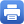Columbia Home
Sep. 26: Ailana Fraser (UBC)

Title: The geometry of extremal eigenvalue problems

Abstract:
When we choose a metric on a manifold we determine the spectrum of the Laplace operator. Thus an eigenvalue may be considered as a functional on the space of metrics. For example the first eigenvalue would be the fundamental vibrational frequency. In some cases the normalized eigenvalues are bounded independent of the metric. In such cases it makes sense to attempt to find critical points in the space of metrics. For surfaces, the critical metrics turn out to be the induced metrics on certain special classes of minimal (mean curvature zero) surfaces in spheres and Euclidean balls. The eigenvalue extremal problem is thus related to other questions arising in the theory of minimal surfaces. In this talk we will give an overview of progress that has been made for surfaces with boundary, and contrast this with some recent results in higher dimensions. This is joint work with R. Schoen.

Wednesday, September 26, 4:30 – 5:30 p.m.

Mathematics 520
Tea will be served at 4:00 p.m.Print this page